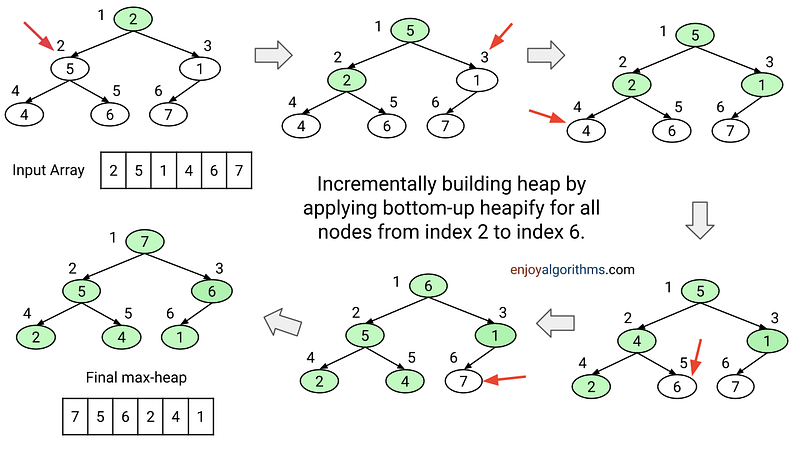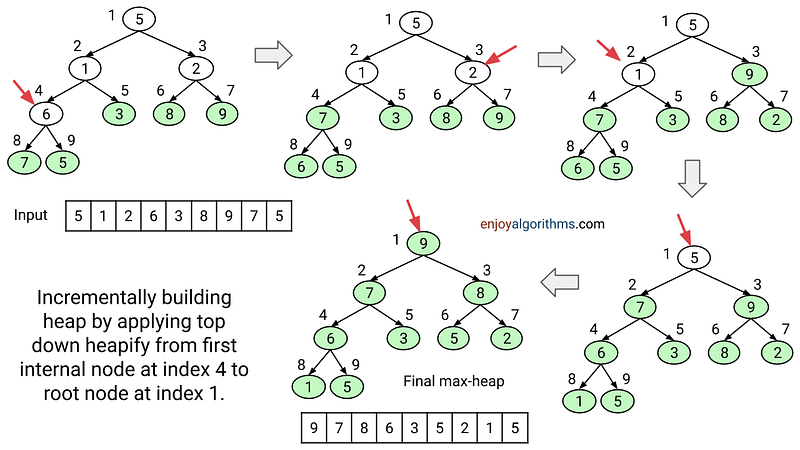# Building Heap from Array

Before performing any max or min heap operations, we need to build a max or min heap from the given array. The critical questions are: How do we achieve this? How do we maintain the heap structure and heap property at each element in the array? We will discuss answers to these questions.

Before discussing the heap-building concept, let’s assume we are using 1-based indexing for the array.

• The root of the heap is at index 1.
• The left child of the ith node is at the (2i)th index, and the right child is at the (2i + 1)th index.
• The parent of the ith node is at the i/2 index.

Furthermore, we use the heapify operation to maintain the heap property at any ith node of the heap. In other words, if there is a violation of the heap property at the ith node, we can call the heapify operation on that node to restore the heap property.

The idea to build min and max heap will be similar: We need to maintain min-heap property at each node in min-heap and max-heap property in max-heap. Here we will discuss the idea to build max-heap.

### Top-down approach to build heap

#### Solution idea

Suppose the heap size is 1 initially i.e. we are assuming that the first element at index 1 is a heap of size 1 and other elements are not part of the heap. Now we traverse the array from the 2nd element and build the heap incrementally by adding each element to the partial heap. By the end of the traversal, our goal would be to create the max heap of size n. How does this idea work? Let’s think!

Suppose we have incrementally created the heap in the same array for the first i elements from index 1 to i, i.e., heap size is equal to i. Now our goal is to include (i + 1)th element in this partial heap. For this, we need to fix the heap property in the down-to-up path from (i + 1)th element to the root node at index 1.

So we compare the (i + 1)th element with its parent node. If the parent node is less than (i + 1)th element, we swap both values. Now, we move to the parent node and do the same process. Overall, we keep moving up until we place the value of (i + 1)th element to its correct position in the max-heap structure. This is a bottom-up heapify process.

Now we have a heap of size i + 1. In the same iterative way, we move to the (i + 2) index and do the same process to add that element to the partial heap, and so on. By the end of this process, we have a heap of size n ready in the same array.#### Solution code C++

``````void bottomUpMaxHeapify(vector<int>& arr, int index)
{
int parent = index / 2;
while (parent >= 1 && arr[parent] < arr[index])
{
swap(arr[parent], arr[index]);
index = parent;
parent = index / 2;
}
}

void buildMaxHeap(vector<int>& arr)
{
for (int i = 2; i <= arr.size() - 1; i = i + 1)
bottomUpMaxHeapify(arr, i);
}``````

#### Solution code Python

``````def bottom_up_max_heapify(arr, index):
parent = index // 2
while parent >= 0 and arr[parent] < arr[index]:
arr[parent], arr[index] = arr[index], arr[parent]
index = parent
parent = index // 2

def build_max_heap(arr):
for i in range(1, len(arr)):
bottom_up_max_heapify(arr, i)``````

#### Time complexity analysis

For the convenience of the analysis, suppose each level of the heap is completely full and there are k number of levels from 0 to k — 1. If the total number of elements in the heap is n, then:

=> n = 2^0 + 2^1 + 2^2 + …. + 2^(k — 2) + 2^(k — 1) = 2^k — 1

=> n ~ 2^k and k ~ logn.

Now think about the worst case! In the worst case, each element will move from its position to the root index 1. So we calculate the total number of operations level by level and do the sum.

At the last level k — 1, there will be 2^(k — 1) number of elements. In the worst case, all these elements will move from level k — 1 to level 0 and perform c(k — 1) number of operations, here c is a constant. Similarly, there will be 2^(k — 2) number of elements at level k — 2 and all these elements will perform c(k — 2) number of operations. And so on.

Total number of operations in the worst-case

= 2^(k — 1)*c(k — 1) + 2^(k — 2)*c(k — 2) + ….. + 2^0*c

= n/2*c(logn — 1) + n/4*c(logn — 2) + …. + c (as we know n ~ 2^k, k ~ logn)

≤ c (n/2*logn + n/4*logn + … + logn)

≤ cn*logn (1/2 + 1/4 + 1/8 + … + 1/n)

≤ cn*logn (1/2 + 1/4 + 1/8 + … infinity)

= cn*logn*(1/2)/(1 – 1/2) [From the sum of infinite geometric series].

= cn*logn = O(nlogn).

So the tight upper bound of the building heap using top-down approach is O(nlogn). This is not surprising because almost n/2 elements are present closer to the leaf node and these elements will take O(logn) time in the worst case. On the other side, a very small number of nodes are closer to the root node and it will take almost constant time. Overall, time complexity is dominated by elements closer to the leaf node.

We are building the heap in the same array, so space complexity = O(1). Now critical questions are: Can we improve the time complexity further? Can we build a heap in place using O(n) time? Yes, we can do this. The idea is a bottom-up approach to heap building!

### Efficient approach: Bottom up approach to build heap

#### Solution idea

This is one of the simplest and smartest algorithms in data structures. The idea is to start from the bottom and apply the top-down heapify procedure at all internal nodes! Let’s understand this in detail.

The elements in the heap from index n/2 + 1 to n are all leaf nodes and a small heap of size 1. So we simply skip the smaller heaps of size 1 and apply the top-down heapify process on all the remaining nodes from index n/2 to 1.

In other words, this process establishes the heap order iteratively. We proceed from right to left from index n/2 to 1 and make the smaller heaps as we go. The idea is that every position in the array is the root of a small heap. So before applying the top-down heapify process to each node, this algorithm ensures that both children of the current node are heap as well. There could be a chance of violation of heap property at the current index, so we can easily apply the top-down heapify procedure to fix this.#### Solution code C++

``````void topDownMaxHeapify(vector<int>& arr, int heapSize, int index)
{
int leftChild = 2 * index;
int rightChild = 2 * index + 1;
int largest = index;

if (leftChild <= heapSize && arr[leftChild] > arr[largest])
largest = leftChild;

if (rightChild <= heapSize && arr[rightChild] > arr[largest])
largest = rightChild;

if (largest != index)
{
swap(arr[index], arr[largest]);
topDownMaxHeapify(arr, heapSize, largest);
}
}

void buildMaxHeap(vector<int>& arr)
{
int heapSize = arr.size() - 1;

for (int i = heapSize / 2; i >= 1; i = i - 1)
topDownMaxHeapify(arr, heapSize, i);
}``````

#### Solution code Python

``````def top_down_max_heapify(arr, heap_size, index):
left_child = 2 * index
right_child = 2 * index + 1
largest = index

if left_child <= heap_size and arr[left_child] > arr[largest]:
largest = left_child

if right_child <= heap_size and arr[right_child] > arr[largest]:
largest = right_child

if largest != index:
arr[index], arr[largest] = arr[largest], arr[index]
top_down_max_heapify(arr, heap_size, largest)

def build_max_heap(arr):
heap_size = len(arr) - 1

for i in range(heap_size // 2, 0, -1):
top_down_max_heapify(arr, heap_size, i)``````

#### Time complexity analysis

This is one of the best algorithms to understand the idea of time complexity analysis. If we observe, we are calling the top-down heapify procedure n/2 times, and each call will take O(log n) time. So, the overall time complexity looks like O(n log n). Although this upper bound is correct, it is not asymptotically tight. The correct upper bound is O(n). How? Let’s think!

If we observe closely, the time for top-down heapify varies with the height of the node in the tree, and the heights of most nodes are small. In other words, the heapify algorithm spends the majority of its time working on small heaps.

For example, to build a heap of 127 elements, we process 32 heaps of height 1, 16 heaps of height 2, 8 heaps of height 3, 4 heaps of height 4, 2 heaps of height 5, and 1 heap of height 6. So, the total number of swapping operations during the heapify process (in the worst case) = 32·1 + 16·2 + 8·3 + 4·4 + 2·5 + 1·6 = 120. Note: The total number of comparisons will be 2 times the total number of swaps. Explore and think!

Suppose there are n nodes, and each heap level is completely full. Here, the comparison of node values is a critical operation. So, for the analysis, we will count the total number of comparison operations in the worst case.

• Here, n/2 nodes are heaps of height 0, and we do not need to perform any operations on these nodes.
• Similarly, n/4 nodes are heaps of height 1, and for each heap, we perform 2 comparison operations in the worst case. The count of comparison operations for these n/4 nodes is n/4 * 2.
• Likewise, n/8 nodes are heaps of height 2, and for each heap, we perform 4 comparison operations in the worst case. The count of comparison operations for these n/8 nodes is n/8 * 4.

And so on. To calculate the total number of comparison operations, we add the operations for all different heights. The total number of comparison operations = n/4 * 2 + n/8 * 4 + n/16 * 6 + … + 1 * 2logn = 2n(1/4 + 2/8 + 3/16 + 4/32 + … + 1/n * logn) ≤ 2n(1/4 + 2/8 + 3/16 + 4/32 + … to infinity).

Suppose S = 1/4 + 2/8 + 3/16 + 4/32 + … till infinity, then S/2 = 1/8 + 2/16 + 3/32 + …till infinity. If we subtract both S and S/2, we will get:

=> S/2 = 1/4 + 1/8 + 1/16 + …..till infinity.

=> S = 1/2 + 1/4 + 1/8 + …..till infinity = (1/2)/(1 – 1/2) = 1 [From the sum of infinite geometric series].

=> So, S = 1.

Total number of comparison operations ≤ 2n (1/4 + 2/8 + 3/16 + 4/32 + … till infinity) = 2n*S = 2n. So the upper bound of the total number of comparisons performed by the bottom-up approach of heap building is 2n. Amazing! So time complexity = O(n). We are using constant extra space, so space complexity = O(1).

We will keep adding more insights to this blog. Enjoy learning, enjoy algorithms! If you have some queries or feedback, please share in the message below!

☆ 16-Week Live DSA Course
☆ 10-Week Live DSA Course